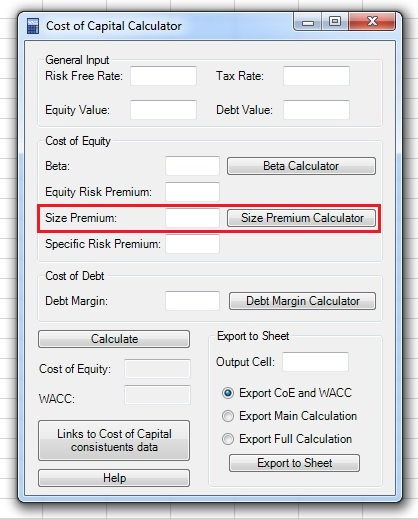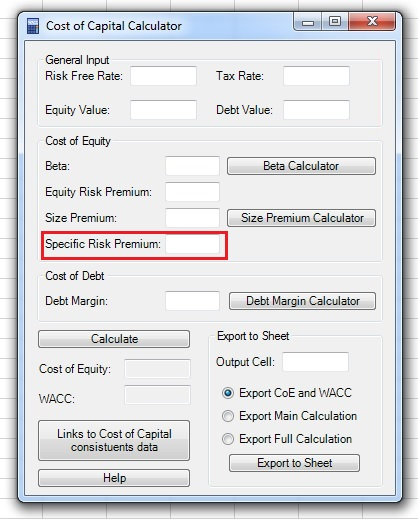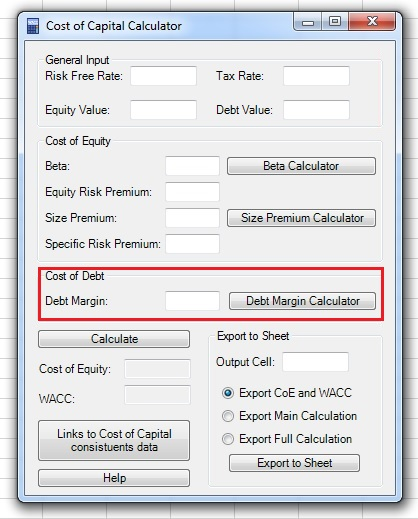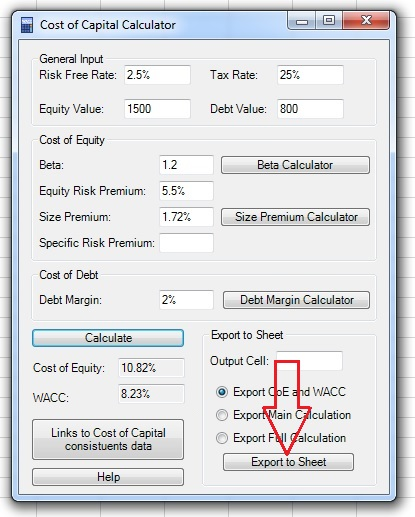This is the Cost of Capital Calculator tutorial.

You can watch the tutorial video, read the step by step text tutorial or go through the step by step screenshots tutorial

##### Step by Step Text Tutorial:

1. Click on the Cost of Capital Calculator icon in the ADD-INS toolbar. The Cost of Capital Calculator window will open.

2. Input the general input parameters:

• Risk Free Rate: Average equity duration is estimated between 17 to 22 years, the risk free rate should be of the same duration.
• Tax Rate: The effective tax rate of the company.
• Equity Value: Estimated equity value of the company.
• Debt Value: Estimated debt value of the company.

3. Input the Beta of the company. If you want, you can use the Beta Calculator to calculate the beta.

4. Input the Equity Risk Premium. You can use the “Links to Cost of Capital constituents data” button to access data sources for Equity Risk Premium.

5. If you want, input the Size premium. You can also use the

6. If you want, you can add a Specific Risk Premium. The specific risk premium is added when you decide that the company bears a specific risk which is not captured by the Beta and Size Premium.

7. If you want to calculate the Weighted Average Cost of Capital (WACC), input the Debt Margin of the company. You can use the Debt Margin Calculator.

8. Click on the “Calculate” button and the Cost of Equity, and, if you entered the Debt margin, also the WACC, will appear in the appropriate fields.

9. Select the first output cell by clicking on the “Output Cell” field, clicking on the cell in the spreadsheet and returning to the Cost of Capital Calculator window.

Note: If the range input field is not empty, you need to delete existing text before selecting a new range in the spreadsheet.

10. Choose the output:

• Export CoE and WACC: exports only the Cost of Equity and WACC values.
• Export Main Calculation: exports the Cost of Equity and WACC values and all the inputs of the main window (note that the Cost of Equity and WACC output is a formula which uses the cells with the input data).
• Export Full Calculation: exports the Cost of Equity and WACC values, all the inputs of the main window and additional input of the Beta, Size premium and Debt Margin calculators, if you used them.

11. Click on the “Export to Sheet” button to export the data.

##### Step by Step Screenshots Tutorial:

1.Click on the Cost of Capital Calculator icon in the ADD-INS toolbar. The Cost of Capital Calculator window will open.

2. Input the general input parameters:

• Risk Free Rate: Average equity duration is estimated between 17 to 22 years, the risk free rate should be of the same duration.
• Tax Rate: The effective tax rate of the company.
• Equity Value: Estimated equity value of the company.
• Debt Value: Estimated debt value of the company.3. Input the Beta of the company. If you want, you can use the Beta Calculator to calculate the beta:4. Input the Equity Risk Premium. You can use the “Links to Cost of Capital constituents data” button to access data sources for Equity Risk Premium.5. If you want, input the Size premium. You can also use the Size Premium Calculator:6. If you want, you can add a Specific Risk Premium. The specific risk premium is added when you decide that the company bears a specific risk which is not captured by the Beta and Size Premium:7. If you want to calculate the Weighted Average Cost of Capital (WACC), input the Debt Margin of the company. You can use the Debt Margin Calculator:8. Click on the “Calculate” button and the Cost of Equity, and, if you entered the Debt margin, also the WACC, will appear in the appropriate fields:9. Select the first output cell by clicking on the “Output Cell” field, clicking on the cell in the spreadsheet and returning to the Cost of Capital Calculator window:

Note: If the range input field is not empty, you need to delete existing text before selecting a new range in the spreadsheet.10. Choose the output:

• Export CoE and WACC: exports only the Cost of Equity and WACC values.
• Export Main Calculation: exports the Cost of Equity and WACC values and all the inputs of the main window (note that the Cost of Equity and WACC output is a formula which uses the cells with the input data).
• Export Full Calculation: exports the Cost of Equity and WACC values, all the inputs of the main window and additional input of the Beta, Size premium and Debt Margin calculators, if you used them.11. Click on the “Export to Sheet” button to export the data: